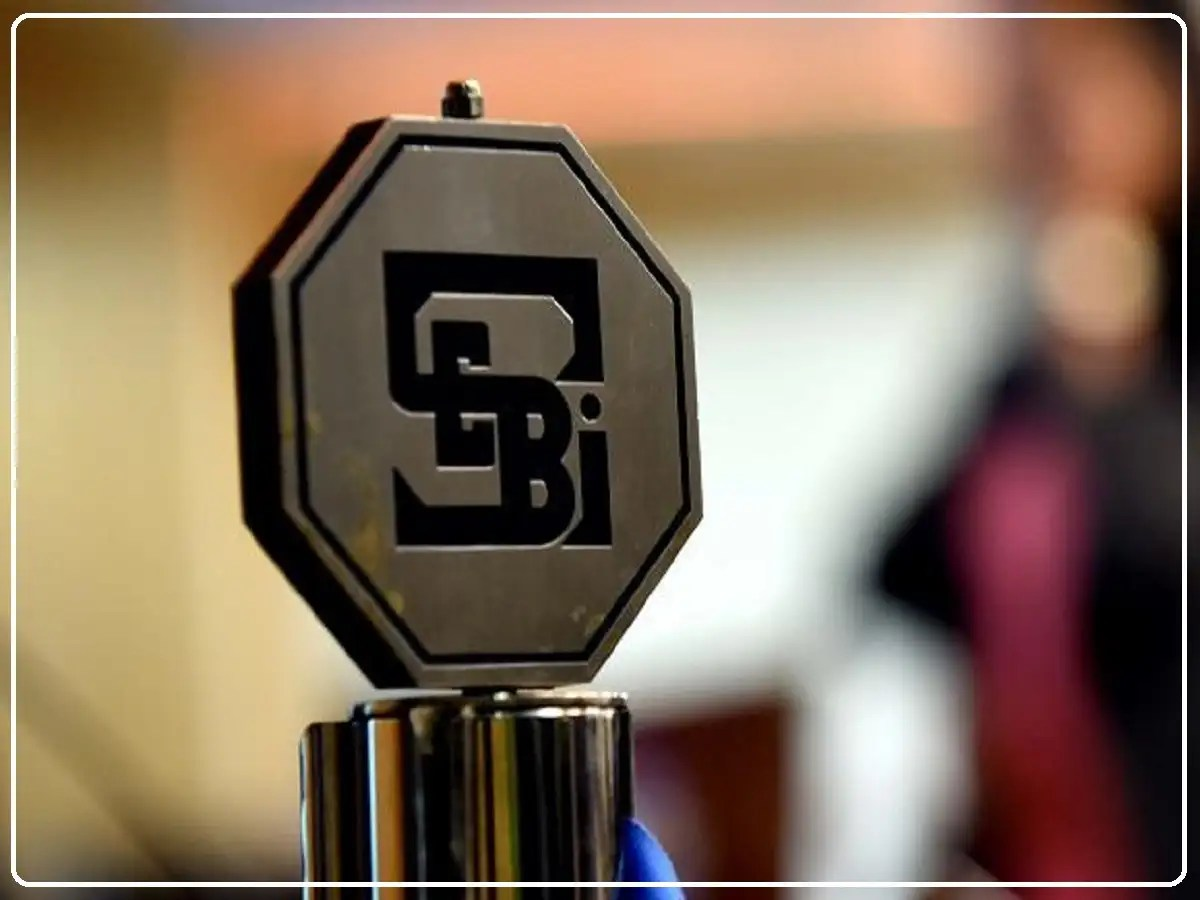CBSE 12th Physics Board Exam 2020: Paper Analysis, Review, Passing Marks & Latest Updates – Watch Live Video!

A snapshot from CBSE 12th Physics Question Paper 2020

Content from CBSE Class 12 Physics Paper 2020

General Instructions:

(i) This question paper comprises four sections – A, B, C and D.
(ii) There are 37 questions in the question paper. All questions are
compulsory.
(iii) Section A: Q. no. 1 to 20 are very short answer type questions carrying 1 mark each.
(iv) Section B: Q. no. 27 to 27 are short-answer type questions carrying 2 marks each.
(v) Section C: Q. no. 28 to 34 are long-answer type questions carrying 3 marks each.
(vi) Section D:Q. no. 35 to 37 are also long answer type questions carrying 5 marks each.
(vii) There is no overall choice in the question paper. However, an internal choice has been provided in two questions of one mark, two questions of two marks, one question of three marks and all the three questions of five marks. You have to attempt only one of the choices in such
questions.
(viii) However, separate instructions are given with each section and
question, wherever necessary.
(ix) Use of calculators and log tables is not permitted.
(x) You may use the following values of physical constants wherever
necessary.

c = 3 x 108 m

= 6.63 x 10 ‒ 34 Js

e = 1.6 x 10 ‒19 C

mo = 4π × 10 ‒7 T m A ‒ 1

ϵo = 8.85 × 10 ‒ 12 C2 N ‒1 m‒1

1/(4πϵo) = 9 × 109 Nm2 C‒ 2

me = 9.1 × 10 ‒ 31  kg

mass of neutron = 1.675 x 10 ‒27 kg

mass of proton = 1.673 x 10 ‒27 kg

Avogadro’s number = 6.023 x 1023 per gram mole

Boltzmann constant = 1.38 x 10 ‒23 JK‒1

Note: Select the most appropriate option from those given below each question.

1. A biconcave lens of power P vertically splits into two identical plano concave parts. The power of each part will be

(a) 2P

(b) P/2

(c) P

(d) P/√2

2. The power factor of a series LCR circuit at resonance will be

(a) 1/2

(b) &frac12;

(c) 0

(d) 1/√2

3. If photons of frequency v are incident on the surfaces of metals. A & B of threshold frequencies v/2 and v/3 respectively, the ratio of the maximum kinetic energy of electrons emitted from A to that from B is

(a) 2:3

(d)1:3

(c) 3:4

(b) √3:√2

4. The electric flux through a closed Gaussian surface depends upon

(a) Net charge enclosed and permittivity of the medium

(b) Net charge enclosed, permittivity of the medium and the size of the Gaussian surface

(c) Net charge enclosed only

(d) Permittivity of the medium only

5. A charge particle after being accelerated through a potential difference ‘V’ enters in a uniform magnetic field and moves in a circle of radius r. If V is doubled, the radius of the circle will become

(a) 2r

(c) 4r

(c) √2r

(d) r/√2

6. The wavelength and intensity of light emitted by a LED depend upon

(a) forward bias and energy gap of the semiconductor

(b) energy gap of the semiconductor and reverse bias

(c) energy gap only

(d) forward bias only

7. The graph showing the correct variation of linear momentum (p) of a charge particles with its de-Broglie wavelength (λ) is.

.

.

Other important articles related to ongoing CBSE board exam 2020

##### RELATED ARTICLES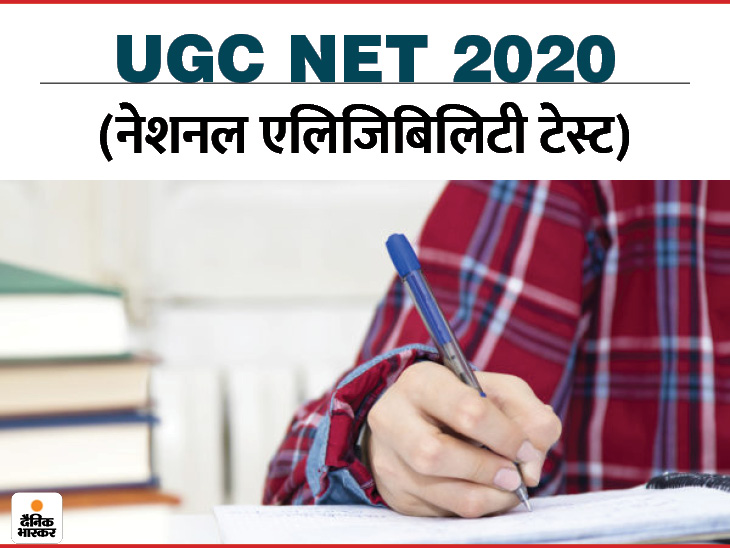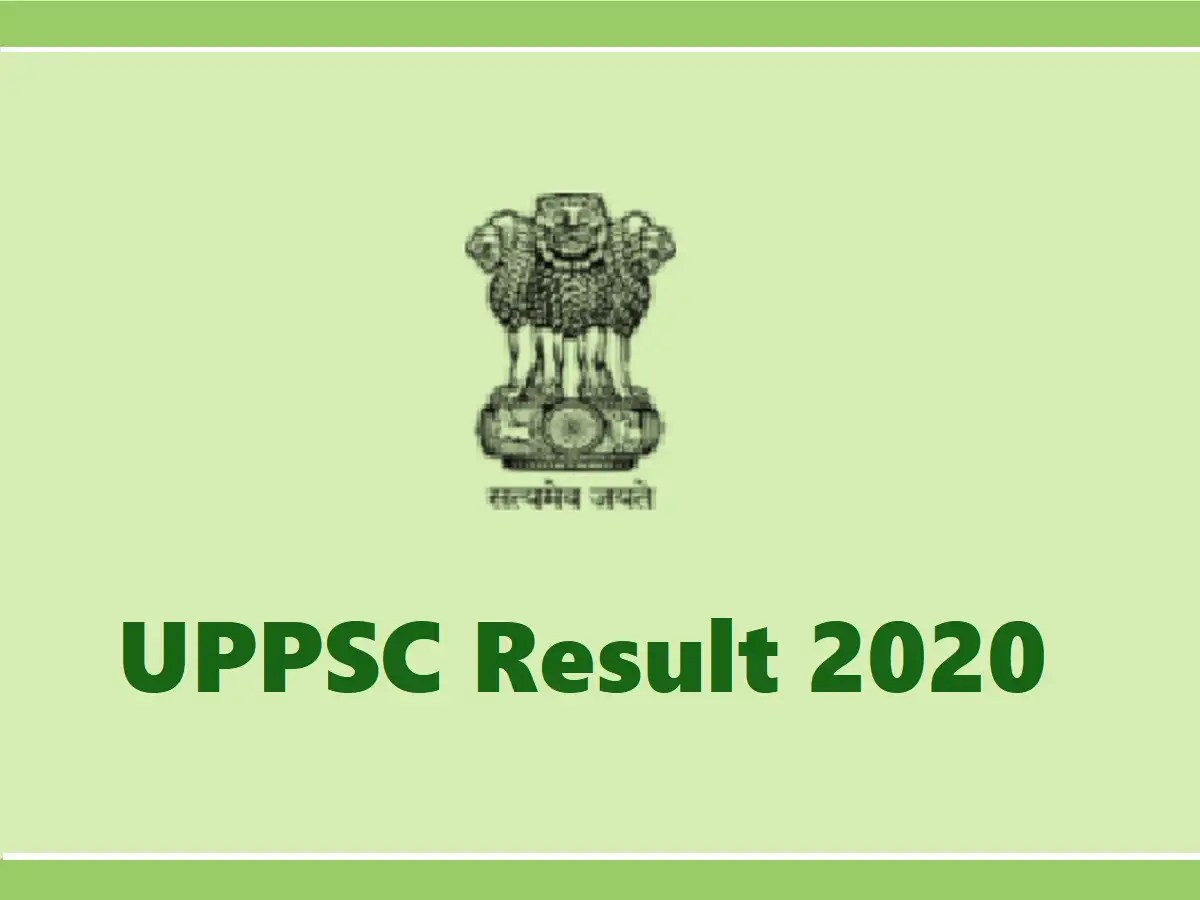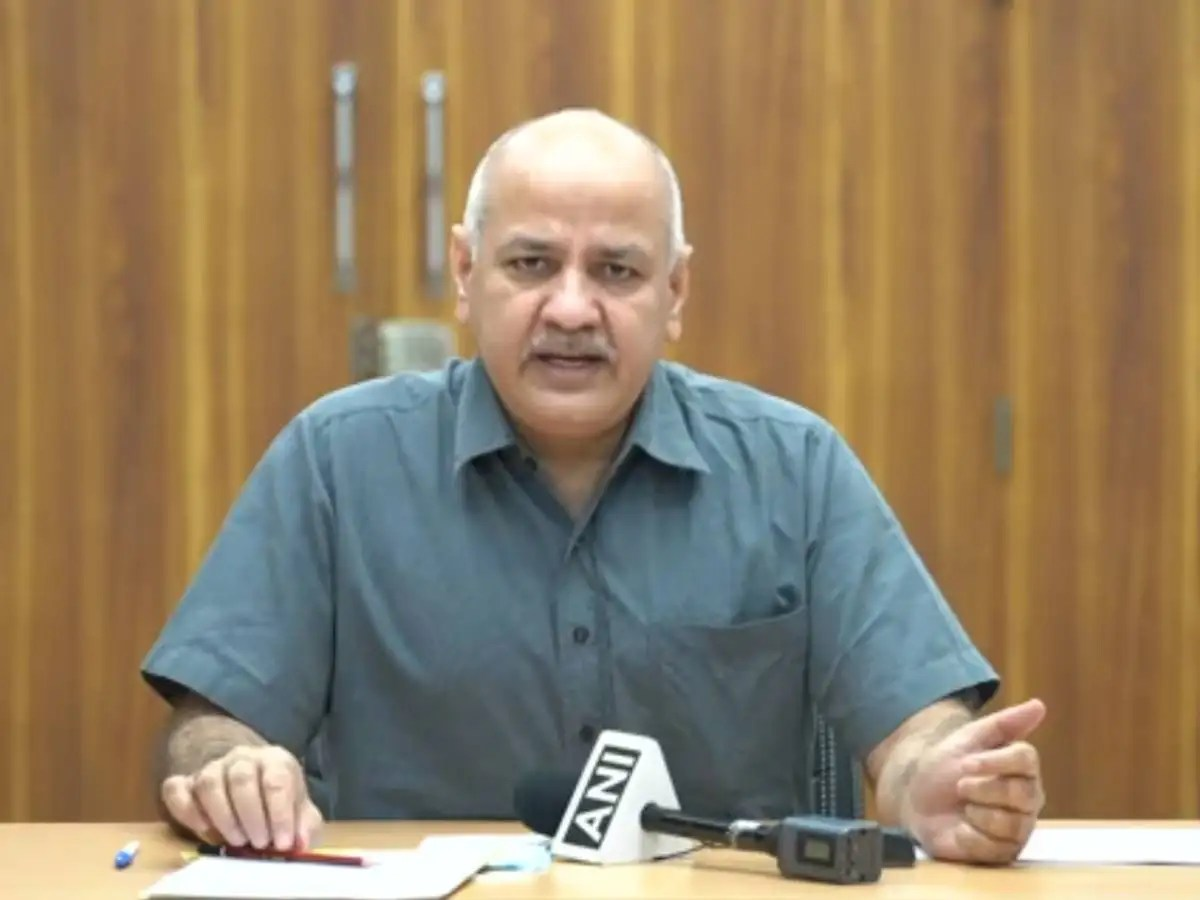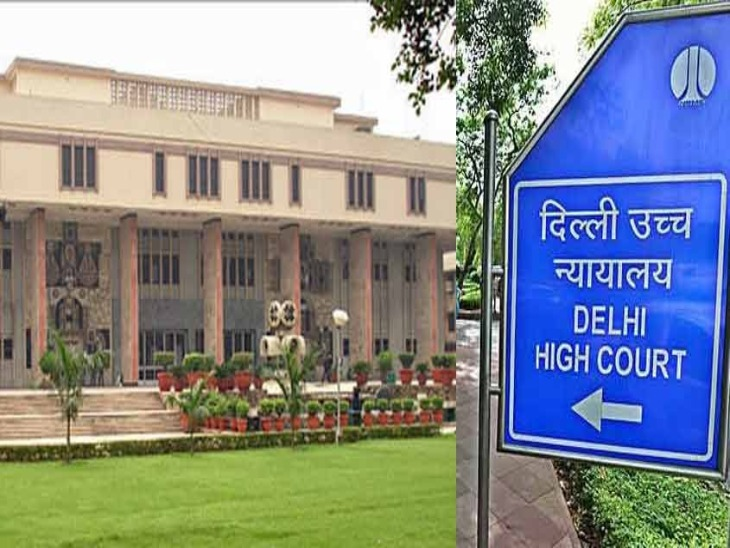##### Latest News